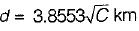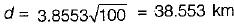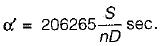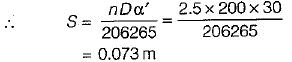Courses

# Levelling - 3

## 10 Questions MCQ Test Topicwise Question Bank for GATE Civil Engineering | Levelling - 3

Description
This mock test of Levelling - 3 for Civil Engineering (CE) helps you for every Civil Engineering (CE) entrance exam. This contains 10 Multiple Choice Questions for Civil Engineering (CE) Levelling - 3 (mcq) to study with solutions a complete question bank. The solved questions answers in this Levelling - 3 quiz give you a good mix of easy questions and tough questions. Civil Engineering (CE) students definitely take this Levelling - 3 exercise for a better result in the exam. You can find other Levelling - 3 extra questions, long questions & short questions for Civil Engineering (CE) on EduRev as well by searching above.
QUESTION: 1

Solution:
QUESTION: 2

### Which one of the following gives the correct distance between the lighthouse and a ship, when the lighthouse whose height is 100 m is visible just above the horizon from the ship?

Solution:

If d is the distance to the visible horizon, it is given by,‘C' being in metres,
∴QUESTION: 3

### Which of the following sights will be applicable for a change point?

Solution:

A change point is the point selected on the route before shifting the instrument. The change point is also called a turning point. The staff is kept on the turning point and a foresight is taken before shifting the instrument. The staff should not be moved when the instrument is being shifted. After the instrument has been shifted and set up at the new location, a back sight is taken on the staff still held at the change point.

QUESTION: 4

Curvature correction to a staff reading in a differential levelling survey is

Solution:
QUESTION: 5

Which one of the following statements is not correct?

Solution:
QUESTION: 6

The bubble tube parallel to the telescope of a theodolite should be more sensitive, since it controls.

Solution:
QUESTION: 7

A line of levels was run from Bench Mark A (770.815) to a Bench Mark B (772.940). The sum of back sights was 32.665 m and sum of fore sights was 30,44 m. The closing error is (in m)

Solution:

RLB = RLA + ∑BS - ∑FS
RLB = 770.815 + 32.665 - 30.44
= 773.035
∴ Closing error = 773.035 - 772.940 = 0.10

QUESTION: 8

The RL of a classroom floor is 30.00 m and the staff reading on the floor is 1.40 m. The staff reading when, held inverted with the bottom touching the Tee beam of the roof is 3.67 m. The height of the beam above the floor is

Solution:

Height = staff reading on floor + inverted staff reading
= 1.40 + 3.67 = 5.07 m

QUESTION: 9

The distance of visible horizon from a height of 36 m above mean sea level is given by

Solution:
QUESTION: 10

If sensitivity of the bubble tube is 30" per 2 mm division then what would be the error in staff reading on a vertically held staff at a distance of 200 m when its bubble is out of centre by 2.5 divisions.

Solution:

The sensitivity of a bubble tube is given by